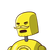# A plane is flying at the height of 5200 m above sea level. At a particular point, it is exactly above a submarine floating 1300 m

A plane is flying at the height of 5200 m above sea level. At a particular point, it is exactly above a submarine floating 1300 m below sea level.Find the vertical distance between them.

3900

5200

6500

7200​

### 2 thoughts on “A plane is flying at the height of 5200 m above sea level. At a particular point, it is exactly above a submarine floating 1300 m”

1.Given:

• Height of plane above the sea level ,h = 5200m
• Depth of submarine floating in the sea ,d = 1300m

To Find:

• Vertical distance between them , S ?

Solution:

As we have to calculate the vertical distance between submarine & plane .

As the submarine is floating 1300m below the sea level . Therefore ,

Vertical distance = Height of plane flying +floating of submarine below sea level.

substitute the value we get

[tex]\longrightarrow[/tex] S = 5200m + 1300m

[tex]\longrightarrow[/tex] S = 6500m

• Hence, the vertical distance between submarine and plane is 6500m .
• The required answer is option (3) 6500m .
2.### Given :-

• A plane is flying at a height of 5200 m above a submarine floating 1300 m below sea level.

### To Find :-

• What is the vertical distance between them.

### Solution :-

Given :

• Distance of plane from submarine = 5200 m
• Distance of submarine from sea level = 1300 m

We have to find the distance between them :

[tex]\bigstar[/tex] Vertical distance between the flight and submarine = Distance of plane from submarine + Distance of submarine from sea level.

Then, according to the question by using the formula we get,

[tex]\implies \sf Distance\: between\: them =\: 5200\: m +\: 1300\: m\\[/tex]

[tex]\implies \sf Distance\: between\: them =\: (5200 + 1300)m\\[/tex]

[tex]\implies \sf\bold{\red{Distance\: between\: them =\: 6500\: m}}[/tex]

[tex]\therefore[/tex] The vertical distance between the flight and submarine is 6500 m .

Hence, the correct options is option no 3) 6500 m .